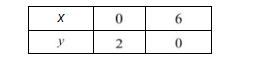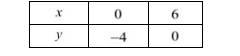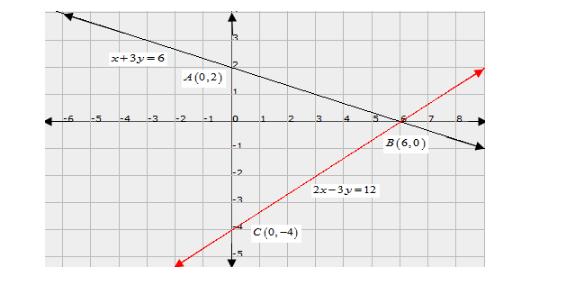# Represent the following pair of equations graphically and write the coordinates of points where the lines intersects y-axis.

Question:

Represent the following pair of equations graphically and write the coordinates of points where the lines intersects y-axis.

$x+3 y=6$

$2 x-3 y=12$

Solution:

The given equations are

$x+3 y=6 \quad \ldots \ldots .(i)$

$2 x-3 y=12 \quad \ldots \ldots . .(i i)$

Putting $x=0$ in equation (i) we get:

$\Rightarrow 0+3 y=6$

$\Rightarrow y=2$

$x=0, \quad y=2$

Putting $y=0$ in equation $(i)$ we get:

$\Rightarrow x+3 \times 0=6$

$\Rightarrow x=6$

$x=6, \quad y=0$

Use the following table to draw the graph.The graph of $(i)$ can be obtained by plotting the two points $A(0,2), B(6,0)$.

$2 x-3 y=12$

Putting $x=0$ in equation (ii) we get:

$\Rightarrow 2 \times 0-3 y=12$

$\Rightarrow y=-4$

$x=0, \quad y=-4$

Putting $y=0$ in equation (ii) we get:

$\Rightarrow 2 x-3 \times 0=12$

$\Rightarrow x=6$

$x=6, \quad y=0$

Use the following table to draw the graph.Draw the graph by plotting the two points $C(0,-4), D(6,0)$ from table.Graph of lines represented by the equations $x+3 y=6,2 x-3 y=12$ meet $y$-axis at $A(0,2), C(0,-4)$ respectively.